# Tidy geographic data

with sf, dplyr, ggplot2, geos and friends

These materials were created for the OpenGeoHub Summer School 2023.

They can be used with reference to the accompanying slides, available at ogh23.robinlovelace.net/opengeohub2023.

See the parent repo and session description in the agenda for context.

# Abstract

This lecture will provide an introduction to working with geographic data using R in a ‘tidy’ way. It will focus on using the `sf` package to read, write, manipulate, and plot geographic data in combination with the `tidyverse` metapackage. Why use the `sf` package with the `tidyverse`? The lecture will outline some of the ideas underlying the `tidyverse` and how they can speed-up data analysis pipelines, while making data analysis code easier to read and write. We will see how the following lines:

``````library(sf)
library(tidyverse)``````

can provide a foundation on which the many geographic data analysis problems can be solved. The lecture will also cover on more recently developed packages that integrate with the `tidyverse` to a greater and lesser extent. We will look at how the `geos` package, which provides a simple and high-performance interface to the GEOS library for performing geometric operations on geographic data, integrates with the `tidyverse`. The `tidyverse` is not the right tool for every data analysis task and we touch on alternatives for working with raster data, with reference to the `terra` package, and alternative frameworks such as `data.table`. Finally, we will also look at how the ‘tidy’ philosophy could be implemented in other programming languages, such as Python.

The focus throughout will be on practical skills and using packages effectively within the wider context of project management tools, integrated development environments (we recommend VS Code with appropriate extensions or RStudio), and version control systems.

# 1 Introduction

## 1.1 Learning objectives

By the end of the session, participants will be able to:

• Read, write, manipulate, and plot geographic data using the `sf` package
• Use the `tidyverse` metapackage to speed-up the writing of geographic data analysis pipelines
• Use the `geos` package to perform geometric operations on geographic data
• Understand the strengths and weaknesses of the `tidyverse` for geographic data analysis

## 1.2 Prerequisites

We recommend you run the code in the practical session with a modern integrated development environment (IDE) such as

• RStudio: an IDE focussed on data science and software development with R. See posit.co for installation instructions.
• VS Code: a general purpose, popular and future-proof IDE with support for R. See github.com/REditorSupport/vscode-R and quarto.org for installation instructions.

After you have installed a suitable IDE you will need to install R packages used in this tutorial. You can install the packages we’ll use with the following commands:

``````# Install remotes if not already installed
if (!requireNamespace("remotes")) {
install.packages("remotes")
}

# The packages we'll use
pkgs = c(
"sf",
"tidyverse",
"geos",
"ggspatial",
"spData"
)``````
``remotes::install_cran(pkgs)``

After running the above commands, you should be able to load the packages with the following command (we will load the packages individually in subsequent sections):

``sapply(pkgs, require, character.only = TRUE)``

# 2 An introduction to geographic data in the tidyverse

The `tidyverse` is a collection of packages that provides a unified set of functions for data science. The name ‘tidyverse’ is a reference to the ‘tidy data’ concept, which means simple data that is in the form of one observation per row and one variable per column . The meaning has broadened to refer to a way of doing data analysis, that tends to make heavy use of tidyverse packages. Load the `tidyverse` with the following command:

``library(tidyverse)``
``````── Attaching core tidyverse packages ──────────────────────── tidyverse 2.0.0 ──
✔ dplyr     1.1.2     ✔ readr     2.1.4
✔ forcats   1.0.0     ✔ stringr   1.5.0
✔ ggplot2   3.4.3     ✔ tibble    3.2.1
✔ lubridate 1.9.2     ✔ tidyr     1.3.0
✔ purrr     1.0.2
── Conflicts ────────────────────────────────────────── tidyverse_conflicts() ──
ℹ Use the conflicted package (<http://conflicted.r-lib.org/>) to force all conflicts to become errors``````

As shown in the output, the package loads 9 sub-packages. In this tutorial we will focus on

• `dplyr`, which provides convenient functions for manipulating data frames
• `ggplot2`, which provides a powerful and flexible system for creating plots

A good way to understand it is to get started with a small dataset. So let’s load the `sf` package and the `spData` package, which contains the `world` dataset:

``library(sf)``
``Linking to GEOS 3.10.2, GDAL 3.4.1, PROJ 8.2.1; sf_use_s2() is TRUE``
``library(spData)``
``````The legacy packages maptools, rgdal, and rgeos, underpinning the sp package,
which was just loaded, will retire in October 2023.
Please refer to R-spatial evolution reports for details, especially
https://r-spatial.org/r/2023/05/15/evolution4.html.
It may be desirable to make the sf package available;
package maintainers should consider adding sf to Suggests:.
The sp package is now running under evolution status 2
(status 2 uses the sf package in place of rgdal)``````
``````To access larger datasets in this package, install the spDataLarge
package with: `install.packages('spDataLarge',

After loading the packages run the following commands to create an object called countries, containing countries whose centroids are within 200km of the Polish border:

``names(world) # check we have the data``
``````  "iso_a2"    "name_long" "continent" "region_un" "subregion" "type"
 "area_km2"  "pop"       "lifeExp"   "gdpPercap" "geom"     ``````
``````poland = world |>
filter(name_long == "Poland")
world_centroids = world |>
st_centroid()``````
``Warning: st_centroid assumes attributes are constant over geometries``
``````country_centroids = world_centroids |>
st_filter(
poland,
.predicate = st_is_within_distance,
dist = 2e5
)
countries = world |>
filter(name_long %in% country_centroids\$name_long)
countries_df = countries |>
select(name_long, pop, area_km2) |>
st_drop_geometry()``````

Don’t worry about the syntax for now. The important thing is that we now have a data frame with three columns, representing the name, population and area of four countries. We can print out the contents of the data frame by typing its name (this is equivalent to `print(countries_df)`):

``countries_df``
``````# A tibble: 4 × 3
name_long           pop area_km2
* <chr>             <dbl>    <dbl>
1 Poland         38011735  310402.
2 Lithuania       2932367   63831.
3 Slovakia        5418649   47068.
4 Czech Republic 10525347   81208.``````

The output above shows information about each country in a tabular. A feature of the tidyverse is that its default data frame class (the `tibble` which extends base R’s `data.frame` as shown below) prints results in an informative and space-efficient way.

``class(countries_df)``
`` "tbl_df"     "tbl"        "data.frame"``

`ggplot2` is dedicated plotting package that is loaded when you load the `tidyverse`. It has native support for geographic objects, as shown in Figure 1, which shows the output of `plot(countries)` next to the equivalent `ggplot2` code.

``plot(countries)``
``````Warning: plotting the first 9 out of 10 attributes; use max.plot = 10 to plot
all``````
``````countries |>
ggplot() +
geom_sf()``````

A characteristic feature of the tidyverse is the use of the pipe operator. You can use R’s new native pipe operator (`|>`), first available in R 4.1.0, or the magrittr pipe operator (`%>%`).

The pipes chain together functions, making it easier to read and write code. It can be particularly useful when used in combination with RStudio’s intellisense feature, which provides suggestions for column names as you type. Try typing the following in RStudio and hitting Tab with the curso located between the brackets on the final line to see this in action. It will allow you to select the variable you’re interested in without quote marks, using a feature called non-standard evaluation (NSE) .

``````countries_df |>
filter()``````

## 2.1 Reading and writing geographic data

You can read and write a wide range of vector geographic data with `sf`. Save the `countries` object to a file called `countries.geojson` and inspect the result.

``sf::write_sf(countries, "countries.geojson", delete_dsn = TRUE)``

You can read the file in again with `read_sf()` (which returns a ‘tidyverse compliant’ `tibble` data frame) or `st_read()`, as shown below.

``````countries_new1 = sf::read_sf("countries.geojson")
``````Reading layer `countries' from data source
`/home/runner/work/opengeohub2023/opengeohub2023/countries.geojson'
using driver `GeoJSON'
Simple feature collection with 4 features and 10 fields
Geometry type: MULTIPOLYGON
Dimension:     XY
Bounding box:  xmin: 12.24011 ymin: 47.75843 xmax: 26.58828 ymax: 56.37253
Geodetic CRS:  WGS 84``````

For most purposes the two representations are the same, although the ‘tibble’ version’s `print` outpout is slightly different.

``````countries_new1 |>
``````Simple feature collection with 2 features and 10 fields
Geometry type: MULTIPOLYGON
Dimension:     XY
Bounding box:  xmin: 14.07452 ymin: 49.0274 xmax: 26.58828 ymax: 56.37253
Geodetic CRS:  WGS 84
# A tibble: 2 × 11
iso_a2 name_long continent region_un subregion   type  area_km2    pop lifeExp
<chr>  <chr>     <chr>     <chr>     <chr>       <chr>    <dbl>  <dbl>   <dbl>
1 PL     Poland    Europe    Europe    Eastern Eu… Sove…  310402. 3.80e7    77.6
2 LT     Lithuania Europe    Europe    Northern E… Sove…   63831. 2.93e6    74.5
# ℹ 2 more variables: gdpPercap <dbl>, geometry <MULTIPOLYGON [°]>``````
``````countries_new2 |>
``````Simple feature collection with 2 features and 10 fields
Geometry type: MULTIPOLYGON
Dimension:     XY
Bounding box:  xmin: 14.07452 ymin: 49.0274 xmax: 26.58828 ymax: 56.37253
Geodetic CRS:  WGS 84
iso_a2 name_long continent region_un       subregion              type
1     PL    Poland    Europe    Europe  Eastern Europe Sovereign country
2     LT Lithuania    Europe    Europe Northern Europe Sovereign country
area_km2      pop  lifeExp gdpPercap                       geometry
1 310402.33 38011735 77.60244  24347.07 MULTIPOLYGON (((23.48413 53...
2  63831.09  2932367 74.51707  26258.21 MULTIPOLYGON (((26.49433 55...``````

A nice function to explore the differences between the two objects is `waldo::compare()`. It shows that, other than their classes, the two objects are identical:

``waldo::compare(countries_new1, countries_new2)``
```````class(old)`: "sf" "tbl_df" "tbl" "data.frame"
`class(new)`: "sf"                "data.frame"``````

See the full list of file formats that you can read and write with `sf` with the following commands:

``````drvs = sf::st_drivers() |>
as_tibble()
``````# A tibble: 6 × 7
name   long_name                       write copy  is_raster is_vector vsi
<chr>  <chr>                           <lgl> <lgl> <lgl>     <lgl>     <lgl>
1 ESRIC  Esri Compact Cache              FALSE FALSE TRUE      TRUE      TRUE
2 FITS   Flexible Image Transport System TRUE  FALSE TRUE      TRUE      FALSE
3 PCIDSK PCIDSK Database File            TRUE  FALSE TRUE      TRUE      TRUE
4 netCDF Network Common Data Format      TRUE  TRUE  TRUE      TRUE      TRUE
5 PDS4   NASA Planetary Data System 4    TRUE  TRUE  TRUE      TRUE      TRUE
6 VICAR  MIPL VICAR file                 TRUE  TRUE  TRUE      TRUE      TRUE ``````

### 2.1.1 Exercises

1. Re-create the `country_centroids` object, using `world_centroids` and `poland` and inputs, but this time using base R syntax with the `[` operator.

• Bonus: use the `bench::mark()` function to compare the performance of the base R and tidyverse implementation
• Open question: Is this a good thing to benchmark? Why or why not?
1. Inspect the full list of drivers, e.g. with the command `View(drvs)`.
• Which formats are you likely to use and why?
• Bonus: take a look at Chapter 8 of Geocomputation with R for more on reading and writing geographic (including raster) data with R.

## 2.2 Attribute operations with dplyr

dplyr is a large package with many functions for working with data frames. The five key ‘verbs’ described as:

• `mutate()` adds new variables that are functions of existing variables
• `select()` picks variables based on their names.
• `filter()` picks cases based on their values.
• `summarise()` reduces multiple values down to a single summary.
• `arrange()` changes the ordering of the rows.

Let’s take a brief look at each.

``````countries_modified = countries |>
mutate(pop_density = pop / area_km2) |>
select(name_long, pop_density) |>
filter(pop_density > 100) |>
arrange(desc(pop_density))
countries_modified``````
``````Simple feature collection with 3 features and 2 fields
Geometry type: MULTIPOLYGON
Dimension:     XY
Bounding box:  xmin: 12.24011 ymin: 47.75843 xmax: 24.02999 ymax: 54.85154
Geodetic CRS:  WGS 84
# A tibble: 3 × 3
name_long      pop_density                                                geom
<chr>                <dbl>                                  <MULTIPOLYGON [°]>
1 Czech Republic        130. (((15.017 51.10667, 14.57072 51.00234, 14.30701 51…
2 Poland                122. (((23.48413 53.9125, 23.24399 54.22057, 22.7311 54…
3 Slovakia              115. (((22.55814 49.08574, 21.60781 49.47011, 20.88796 …``````

The `summarise()` function is often used in combination with `group_by()`, e.g. as follows:

``````countries_summarised = countries |>
group_by(contains_a = str_detect(name_long, "a")) |>
summarise(n = n(), mean_pop = mean(pop))
countries_summarised``````
``````Simple feature collection with 2 features and 3 fields
Geometry type: POLYGON
Dimension:     XY
Bounding box:  xmin: 12.24011 ymin: 47.75843 xmax: 26.58828 ymax: 56.37253
Geodetic CRS:  WGS 84
# A tibble: 2 × 4
contains_a     n  mean_pop                                                geom
<lgl>      <int>     <dbl>                                       <POLYGON [°]>
1 FALSE          1 10525347  ((15.017 51.10667, 14.57072 51.00234, 14.30701 51.…
2 TRUE           3 15454250. ((26.49433 55.61511, 25.53305 56.1003, 25.00093 56…``````

The operation creates a new variable called `contains_a` that is `TRUE` if the country name contains an “a” and `FALSE` otherwise. Perhaps more impressively, it also automatically updated the geometry column of the combined countries containing the letter “a”, highlighting `dplyr`’s ability to work with geographic data represented as `sf` objects.

``````countries_summarised |>
ggplot() +
geom_sf(aes(fill = contains_a)) +
geom_sf(data = countries, fill = NA, linetype = 3) ``````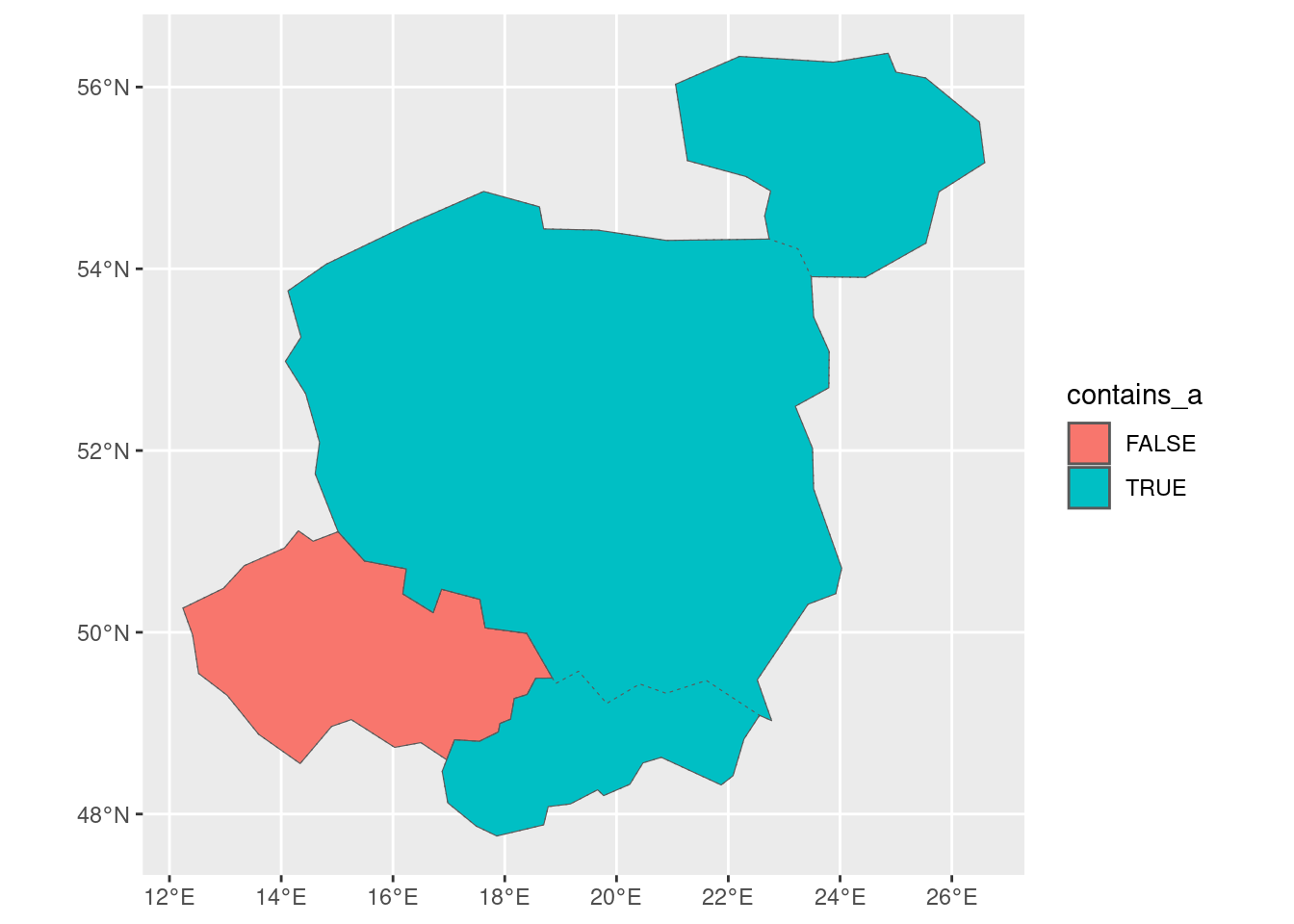Figure 2: Result of running dplyr group_by() and summarise() functions on countries data

### 2.2.1 Exercises

1. Create a new data frame called `countries_modified2` that contains the name, population and area of countries with a population density of more than 100 people per km2, sorted by area in descending order.
2. Do the same with base R functions and the `[` operator.
• What are the pros and cons of each?
• Which do you prefer?

## 2.3 Making maps with ggplot2

As shown above, `geom_sf()` works ‘out of the box’ with geographic data. We can modify plotting commands to control outputs as showing in Figure 3 and generate publishable maps.

``````library(ggspatial)
countries |>
ggplot() +
geom_sf(fill = "grey80", color = "black") +
geom_sf(data = countries_modified, aes(fill = pop_density)) +
scale_fill_viridis_c() +
theme_minimal()``````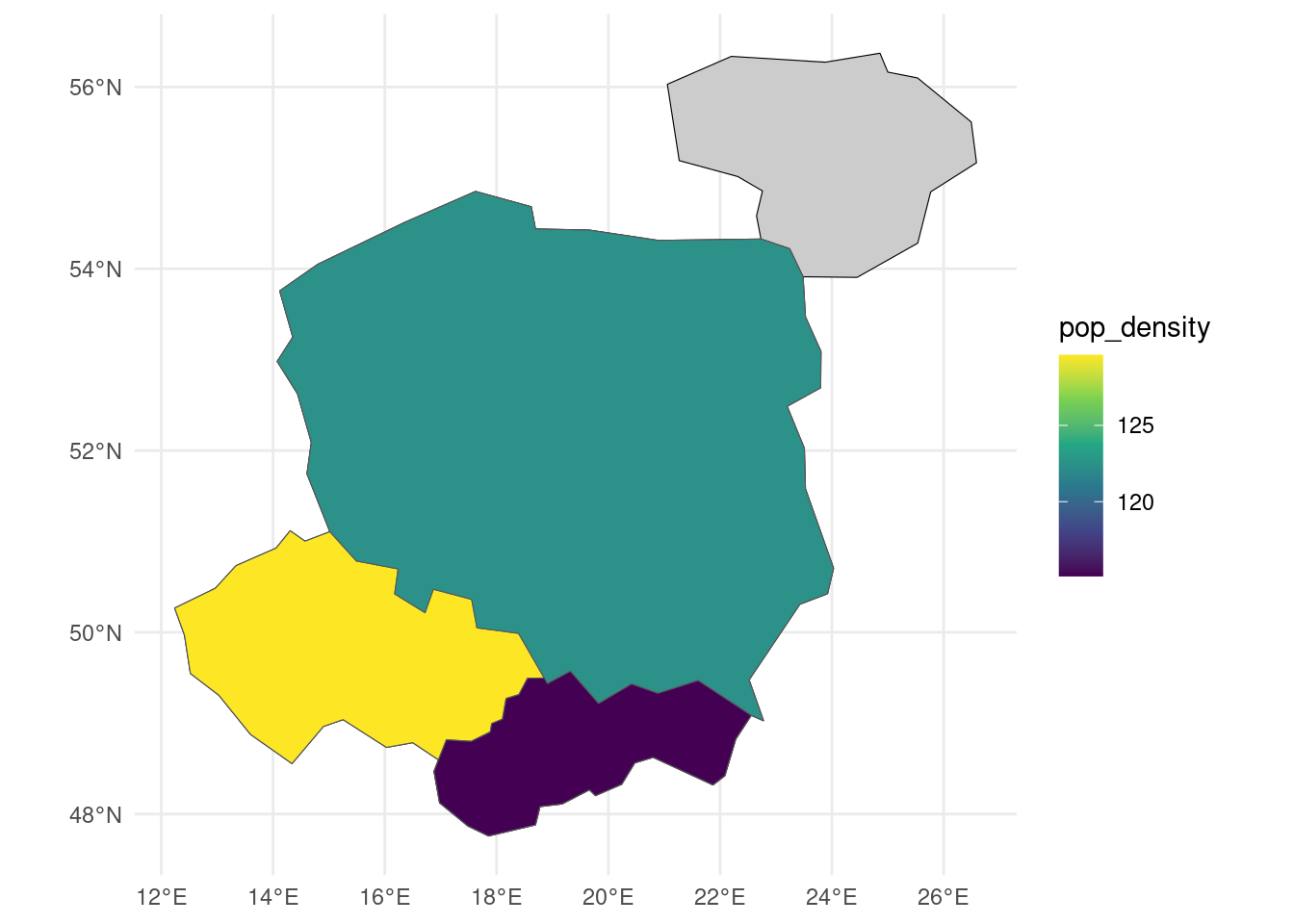Figure 3: Map created with ggplot2, with fill color controlled by the pop_density variable and multiple layers.

Map making is an iterative and time consuming process. Iterate on the code above, e.g. by changing the color palette, adding a title, and adding a legend.

There are many add-ons to `ggplot2`. `ggspatial` can be used to add a basemap to a plot with `annotation_map_tile()`, as illustrated in Figure 4.

``rosm::osm.types()``
``````  "osm"                    "opencycle"              "hotstyle"
 "loviniahike"            "loviniacycle"           "stamenbw"
 "stamenwatercolor"       "osmtransport"           "thunderforestlandscape"
 "thunderforestoutdoors"  "cartodark"              "cartolight"            ``````
``````ggplot() +
annotation_map_tile() +
layer_spatial(countries_modified, aes(fill = pop_density),
linewidth = 3, alpha = 0.3) +
scale_fill_viridis_c()``````
``````
|
|                                                                      |   0%
|
|==================                                                    |  25%
|
|===================================                                   |  50%
|
|====================================================                  |  75%
|
|======================================================================| 100%``````

### 2.3.1 Exercises

1. With reference to the documentation at ggplot2.tidyverse.org/index.html, modify the code above to create a map with a title, legend and a different color palette.
2. With reference to paleolimbot.github.io/ggspatial/, add annotations including scale bar, north arrow and a text label to the map.
3. Bonus: try map making with `tmap` and test out the interactive mode (set with `tmap_mode("interactive")`).”
4. Bonus: try reproducing maps presented in Chapter 9 of Geocomputation with R with `ggplot2` and `ggspatial`. Which mapping framework do you prefer and why?
5. If you use raster data, take a look at the `tidyterra` documentation.

# 3 Data from OSM and data frames

We’ll start this section by creating some data ourselves, representing 4 points of interest (pois) in relation to the OpenGeoHub Summer School 2023.

``````poi_df = tribble(
~name, ~lon, ~lat,
"Faculty",        16.9418, 52.4643,
"Hotel ForZa",    16.9474, 52.4436,
"Hotel Lechicka", 16.9308, 52.4437,
"FairPlayce",     16.9497, 52.4604
)
poi_sf = sf::st_as_sf(poi_df, coords = c("lon", "lat"))
sf::st_crs(poi_sf) = "EPSG:4326"``````
``````# column: screen-inset-shaded
library(leaflet)
leaflet() %>%
lng = poi_df\$lon,
lat = poi_df\$lat,
popup = poi_df\$name
)``````

For comparison, we can create the same map with a single line of code with `{tmap}` (after loading and setting-up the package) as follows:

``````library(tmap)
tmap_mode("view")``````
``tmap mode set to interactive viewing``

We will also check the version of `{tmap}` that we’re using, in anticipation of a major update (to v4, expected later in 2023).

``tm_shape(poi_sf) + tm_bubbles(popup.vars = "name")``

The interactive maps below illustrate the outputs of the preceding map-making commands, highlighting the convenience of using `{tmap}`, which requires far fewer lines of code, although `{leaflet}` and other low-level map-making packages can offer more control.

Turn off the interactive mode as follows.

``tmap_mode("plot")``
``tmap mode set to plotting``

As a taster for the OSM workshop, let’s read-in some data from OSM. As our study area, we’ll use a 500 m buffer around the convex hull of the Faculty and FairPlayce points.

``````pois_buffer = poi_sf |>
filter(str_detect(name, "Fair|Faculty")) |>
st_union() |>
st_convex_hull() |>
st_buffer(dist = 500)``````

We’ll save the output for future reference:

``````pois_buffer_simple = pois_buffer |>
st_simplify(dTolerance = 10)
plot(pois_buffer)
plot(pois_buffer_simple, add = TRUE, border = "blue")``````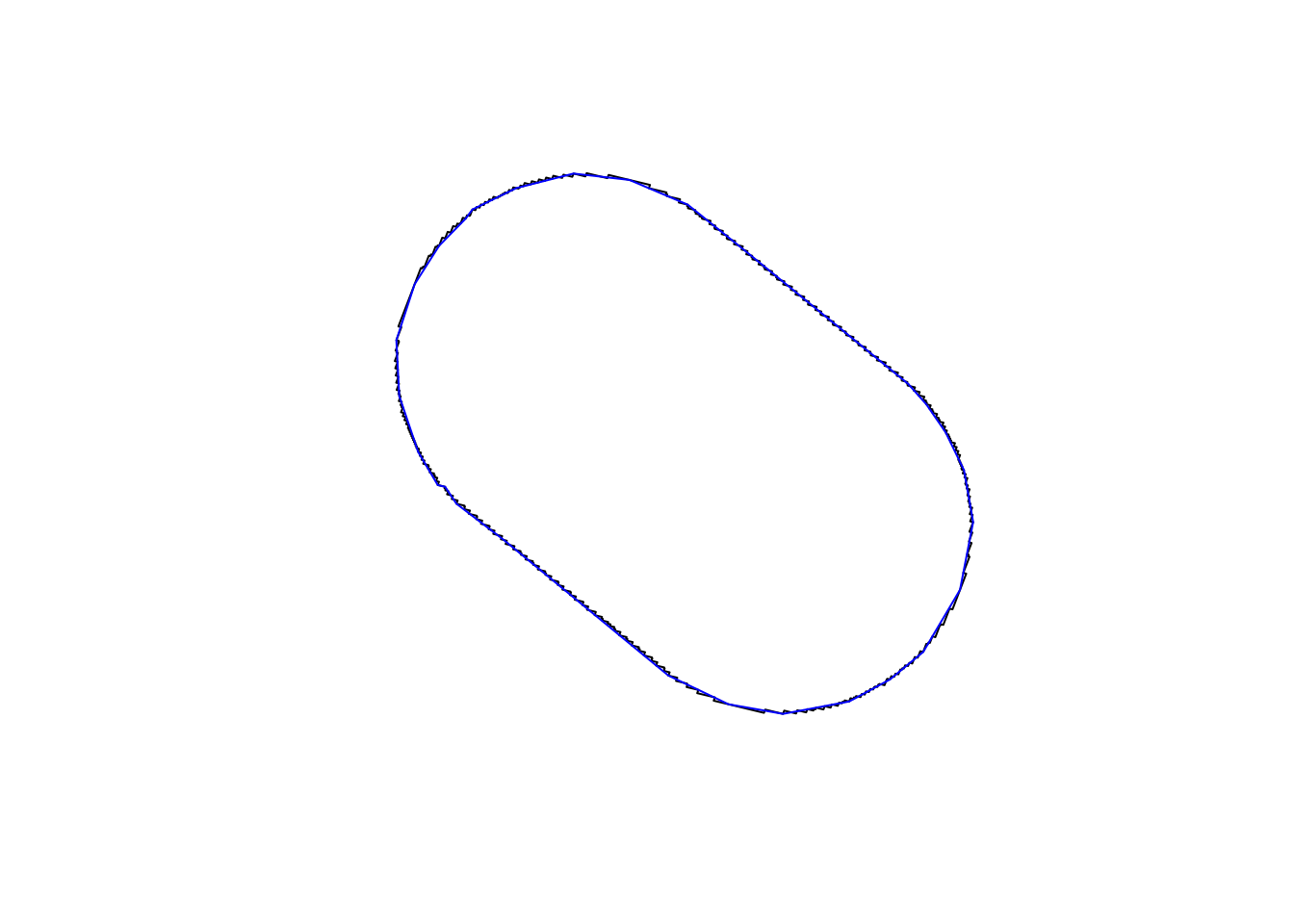``sf::write_sf(pois_buffer_simple, "pois_buffer_simple.geojson", delete_dsn = TRUE)``
``extra_tags = c("maxspeed", "foot", "bicycle")``
``````lines = osmextract::oe_get(
"Poznan",
layer = "lines",
extra_tags = extra_tags,
boundary = pois_buffer,
boundary_type = "clipsrc"
)``````

Filter out only the ones we need as follows:

``````lines_highways = lines |>
filter(!is.na(highway))
plot(lines\$geometry)``````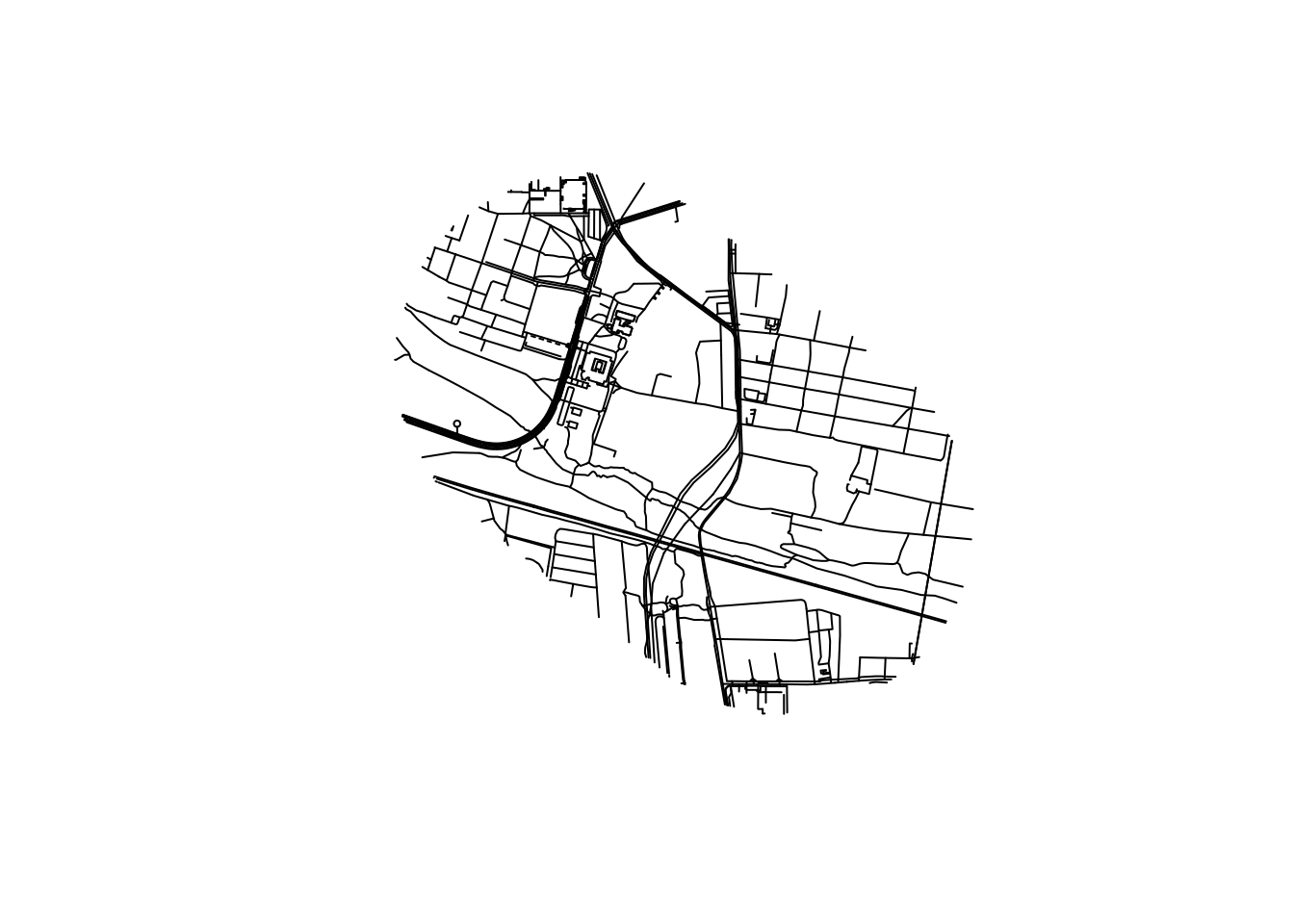``table(lines\$highway)``
``````
footway living_street          path      platform      proposed
83            13            49             8            13
residential       service         steps      tertiary         track
76            67            12             9            10 ``````

Let’s add some polygons to the map, representing the buildings in the area.

``````polygons = osmextract::oe_get(
"Poznan",
layer = "multipolygons",
boundary = pois_buffer,
boundary_type = "clipsrc"
)``````
``````buildings = polygons |>
filter(!is.na(building))
polygons_geog = buildings |>
filter(str_detect(name, "Geog"))``````

Plot the results as follows:

``````m_osm = tm_shape(buildings) +
tm_fill("lightgrey") +
tm_shape(lines_highways) +
tm_lines() +
tm_shape(polygons_geog) +
tm_polygons(col = "red") ``````

You can export the `lines_highways` object to GeoJSON as follows:

``sf::write_sf(lines_highways, "data/lines_highways.geojson")``

Let’s load a GPX file representing a route from the Faculty to FairPlayce.

``````u_gpx = "https://www.openstreetmap.org/trace/9741677/data"
f_gpx = paste0(basename(u_gpx), ".gpx")
sf::st_layers(f_gpx)``````
``````Driver: GPX
Available layers:
layer_name     geometry_type features fields crs_name
1    waypoints             Point        0     23   WGS 84
2       routes       Line String        0     12   WGS 84
3       tracks Multi Line String        1     12   WGS 84
4 route_points             Point        0     25   WGS 84
5 track_points             Point     1525     29   WGS 84``````
``gpx = sf::read_sf(f_gpx, layer = "track_points")``

We can divide the GPS points into n groups of equal length as follows:

``````gpx_mutated = gpx |>
mutate(minute = lubridate::round_date(time, "minute")) |>
mutate(second = lubridate::round_date(time, "second"))
summary(gpx_mutated\$minute)``````
``````                      Min.                    1st Qu.
"2023-08-27 08:02:00.0000" "2023-08-27 08:20:00.0000"
Median                       Mean
"2023-08-27 08:36:00.0000" "2023-08-27 08:38:42.8065"
3rd Qu.                       Max.
"2023-08-27 08:59:00.0000" "2023-08-27 09:14:00.0000" ``````

Let’s create an animated map, as illustrated in Figure 6, with the following code:

``````# ?tmap_animation
m_faceted = m_osm +
tm_shape(gpx_mutated[pois_buffer, ]) +
tm_dots(size = 0.8, legend.show = FALSE) +
tm_facets("second", free.coords = FALSE, ncol = 1, nrow = 1) +
tm_scale_bar()
tmap_animation(m_faceted, delay = 2, filename = "gpx.gif")``````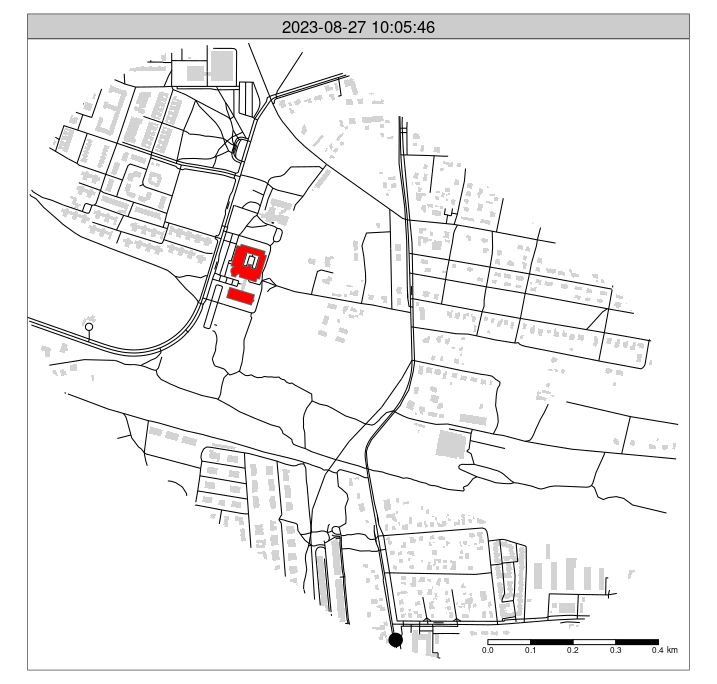Figure 6: Animated map of a GPX file representing a route from central Poznan to FairPlayce.

## 3.2 Exercises

1. Create `sf` objects representing the following features in Poznan (hint: try using functions for geocoding such as `stplanr::geo_code()` or `tmaptools::geocode_OSM()`):
• The main train station
• The airport
2. Identify interesting features in the surrounding area from OSM and plot them in static and interactive maps.

# 4`tidyverse` + `geos`

The `geos` package provides a simple and high-performance interface to the GEOS library for performing geometric operations on geographic data. Load it as follows:

``library(geos)``

`geos` works well with the `tidyverse` and `sf` packages, as shown below.

## 4.1 Finding suitable coordinate reference systems

`geos` is designed to work with projected data, so we will reproject the `countries` object to a different CRS before proceeding.

``````suitable_crs = crsuggest::suggest_crs(countries)
suitable_crs``````
``````# A tibble: 10 × 6
crs_code crs_name                        crs_type crs_gcs crs_units crs_proj4
<chr>    <chr>                           <chr>      <dbl> <chr>     <chr>
1 3328     Pulkovo 1942(58) / GUGiK-80     project…    4179 m         +proj=st…
2 2180     ETRF2000-PL / CS92              project…    9702 m         +proj=tm…
3 3120     Pulkovo 1942(58) / Poland zone… project…    4179 m         +proj=st…
4 2173     Pulkovo 1942(58) / Poland zone… project…    4179 m         +proj=st…
5 2172     Pulkovo 1942(58) / Poland zone… project…    4179 m         +proj=st…
6 2177     ETRF2000-PL / CS2000/18         project…    9702 m         +proj=tm…
7 2178     ETRF2000-PL / CS2000/21         project…    9702 m         +proj=tm…
8 8353     S-JTSK [JTSK03] / Krovak East … project…    8351 m         +proj=kr…
9 8352     S-JTSK [JTSK03] / Krovak        project…    8351 m         +proj=kr…
10 5514     S-JTSK / Krovak East North      project…    4156 m         +proj=kr…``````

We’ll use the second of these, EPSG:2180, after checking (the package’s top suggestion is not always the most up-to-date or appropriate option).

``````crs1 = paste0("EPSG:", suitable_crs\$crs_code)
crs1``````
`` "EPSG:2180"``
``countries_projected = sf::st_transform(countries, crs = crs1)``
``````countries_geos = as_geos_geometry(sf::st_geometry(countries_projected))
countries_geos``````
``````<geos_geometry with CRS=ETRF2000-PL / CS92>
 <MULTIPOLYGON [169518 135742...854986 777315]>
 <MULTIPOLYGON [628064 681310...982752 961090]>
 <MULTIPOLYGON [343349 -11504...759703 189400]>
 <MULTIPOLYGON [18570 83048...489369 371643]>  ``````

The package only deals with geometries: the attribute data is removed when you convert an `sf` object to a `geos` object. You can store `geos` objects in a data frame and still use `dplyr` functions to process them:

``````countries_geos_df = bind_cols(countries_df, geos = countries_geos)
countries_summarised_df = countries_geos_df |>
group_by(contains_a = str_detect(name_long, "a")) |>
summarise(n = n(), mean_pop = mean(pop))
countries_summarised_df``````
``````# A tibble: 2 × 3
contains_a     n  mean_pop
<lgl>      <int>     <dbl>
1 FALSE          1 10525347
2 TRUE           3 15454250.``````

Note: the `geos` column has gone! This is because `geos` columns are not ‘sticky’ like `sf` columns. Let’s see how to get them back.

``````countries_union1 = countries_geos |>
geos_unary_union()
plot(countries_union1)
countries_union2 = countries_geos |>
geos_make_collection() |>
geos_unary_union()
plot(countries_union2)``````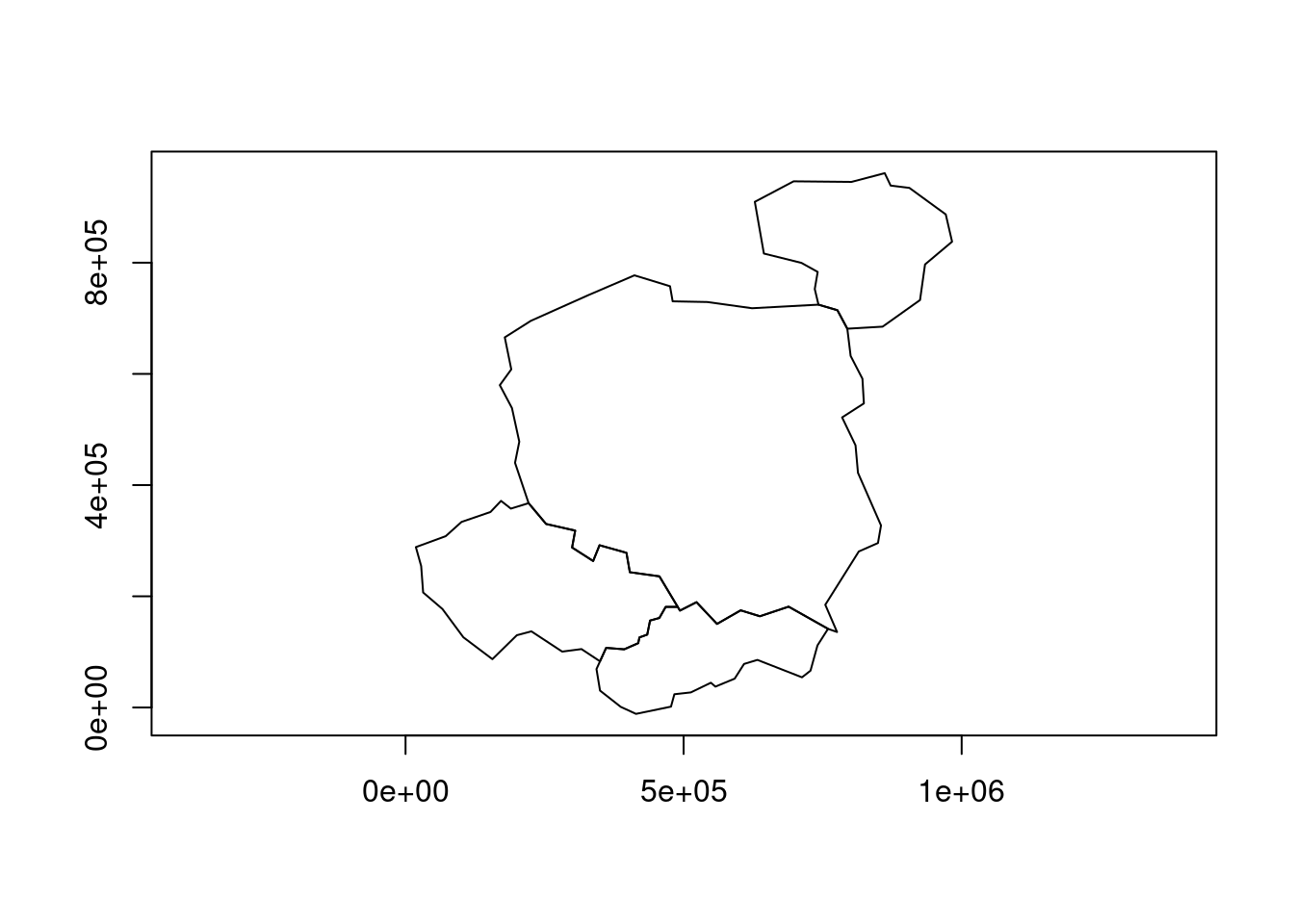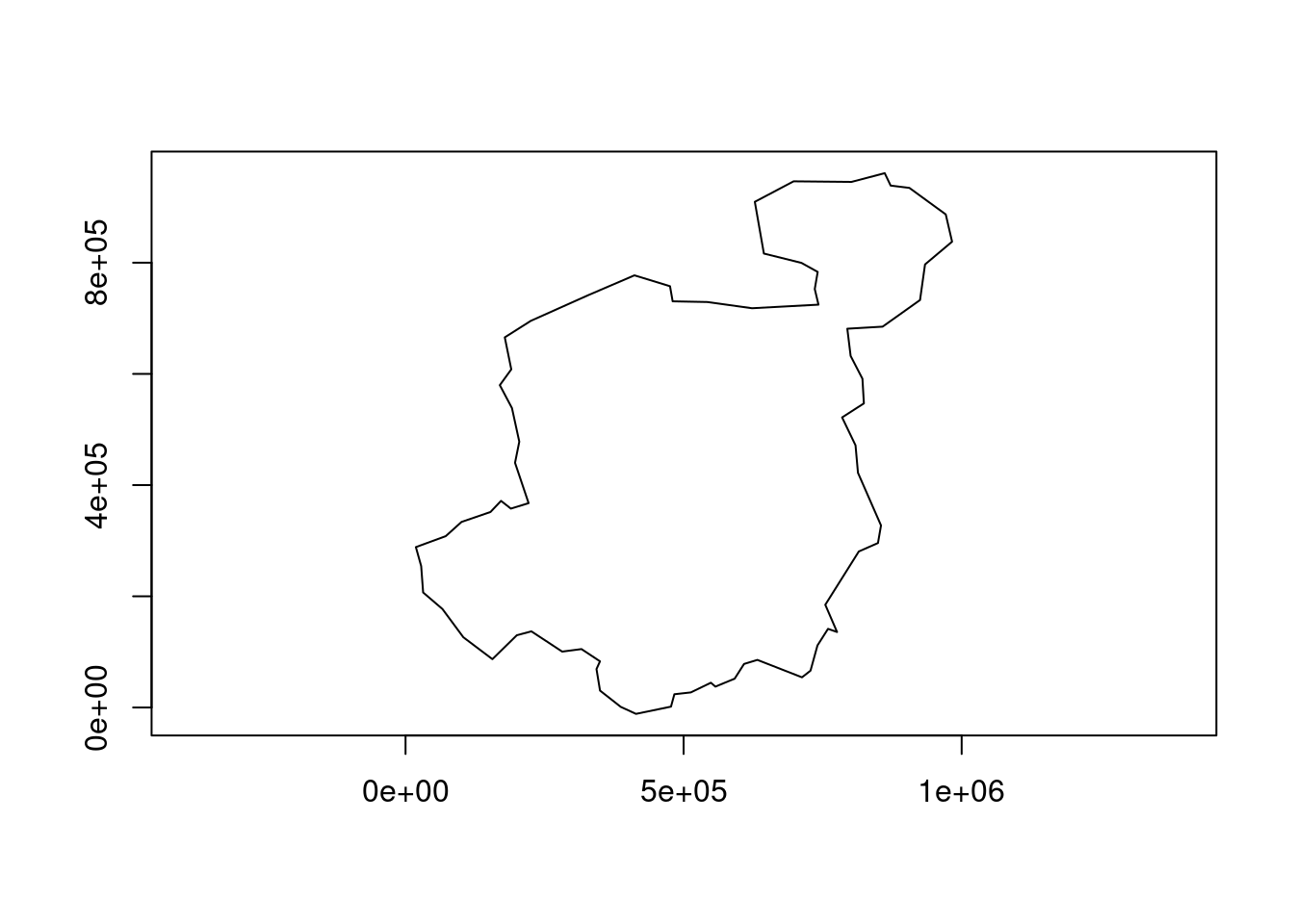However, you can add the union of the grouped columns as follows:

``````countries_summarised_geos = countries_geos_df |>
group_by(contains_a = str_detect(name_long, "a")) |>
summarise(n = n(), mean_pop = mean(pop),
geometry = geos_unary_union(geos_make_collection(geos)))
countries_summarised_geos``````
``````# A tibble: 2 × 4
contains_a     n  mean_pop geometry
<lgl>      <int>     <dbl> <geos_geom>
1 FALSE          1 10525347  <POLYGON [18570 83048...489369 371643]>
2 TRUE           3 15454250. <POLYGON [169518 -11504...982752 961090]>``````
``plot(countries_summarised_geos\$geometry)``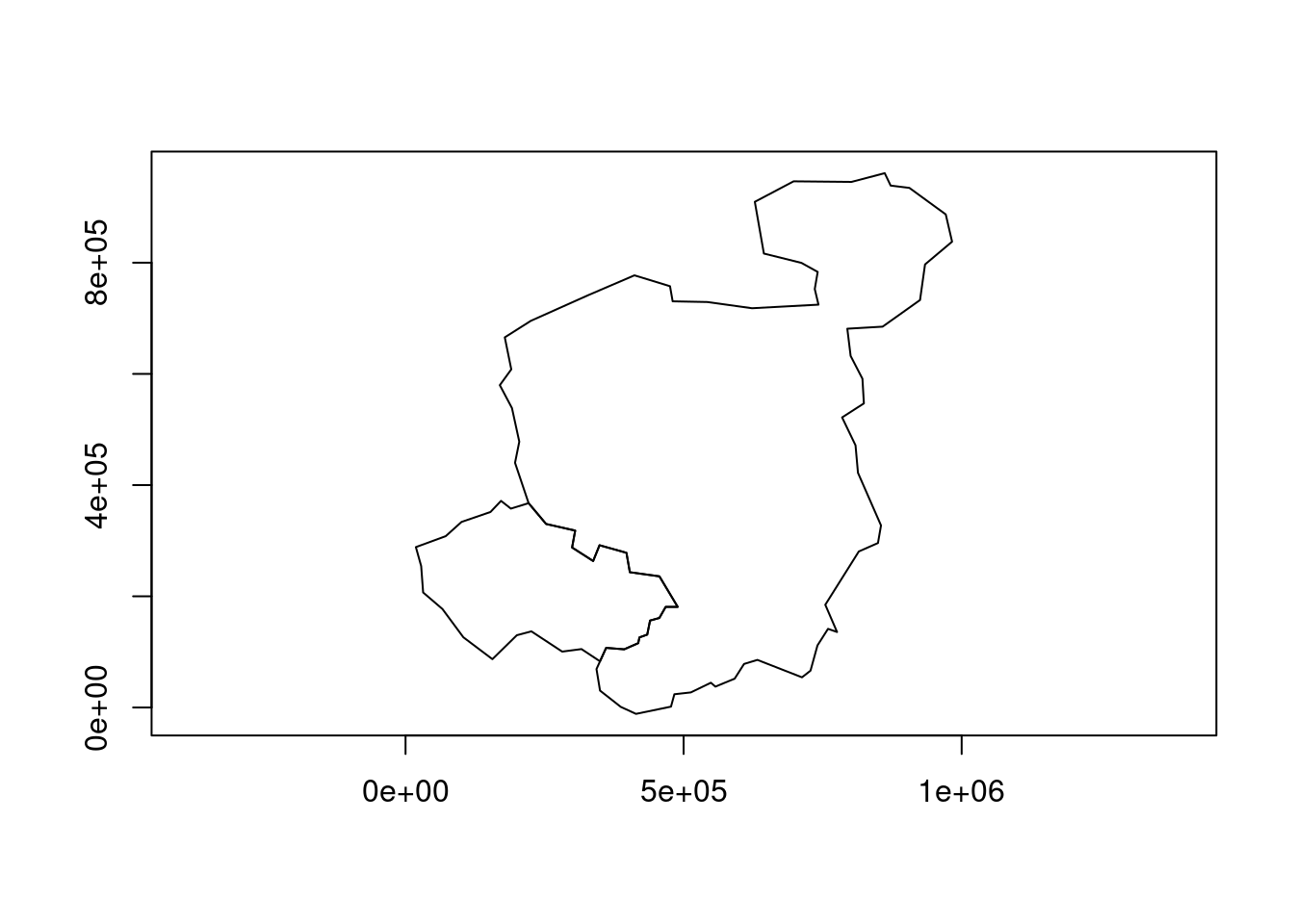Convert back to an `sf` object as follows:

``````countries_summarised_geos_sf = st_as_sf(countries_summarised_geos)
# waldo::compare(
#   countries_summarised,
#   countries_summarised_geos_sf
#   )``````

Aside from geometry names and minor differences in the geometries, the two objects are identical. This raises the question: why use `geos` at all? The answer can be found by following the exercises below.

## 4.2 Exercises

1. Benchmark the union operation in `geos` and `sf` with the `bench::mark()` function.
• Which is faster?
• Which is easier to use?
• Which do you prefer?

# 5 Worked example: data from Poznan

For many people ‘learning by doing’ is the most effective way to pick up new technical skills. So let’s work with some larger datasets. Note: the input data and code below are adapted from Michael Dorman’s tutorial on Working with Spatial Data in Python.

## 5.1 Getting and reading-in the data

To get the data for this session, download and unzip the data.zip file in the releases. You can do that in R with the following commands:

``````u = "https://github.com/Robinlovelace/opengeohub2023/releases/download/data/data.zip"
f = basename(u)
if (!dir.exists("data")) {
unzip(f)
}``````

``list.files("data")[1:3]``
`` "gtfs" "hls"  "osm" ``

## 5.2 Vector data

Let’s start by reading-in a dataset representing transport-related features around Poznan (note: you need to have downloaded and unzipped the `data.zip` file into your project or working directory for this to work):

``````pol_all = sf::read_sf("./data/osm/gis_osm_transport_a_free_1.shp")
pol_all``````
``````Simple feature collection with 282 features and 4 fields
Geometry type: POLYGON
Dimension:     XY
Bounding box:  xmin: 15.76877 ymin: 51.42587 xmax: 18.51031 ymax: 53.52821
Geodetic CRS:  WGS 84
# A tibble: 282 × 5
osm_id    code fclass          name                                  geometry
<chr>    <int> <chr>           <chr>                            <POLYGON [°]>
1 27923283  5656 apron           <NA>                 ((16.84088 52.42479, 16.…
2 28396243  5656 apron           <NA>                 ((16.9675 52.32743, 16.9…
3 28396249  5656 apron           <NA>                 ((16.98029 52.32399, 16.…
4 28396250  5656 apron           <NA>                 ((16.97407 52.32418, 16.…
5 30164579  5656 apron           <NA>                 ((16.71011 53.16458, 16.…
6 32225811  5601 railway_station Czerwonak            ((16.9798 52.46868, 16.9…
7 36204378  5622 bus_station     <NA>                 ((16.95469 52.40964, 16.…
8 50701732  5651 airport         Lądowisko Poznań-Be… ((17.19788 52.53491, 17.…
9 55590985  5622 bus_station     Dworzec PKS-stanowi… ((17.20243 52.80927, 17.…
10 56064358  5651 airport         Port lotniczy Zielo… ((15.76877 52.13175, 15.…
# ℹ 272 more rows``````

Let’s filter-out a feature that matches a particular character string:

``````pol = pol_all |>
filter(str_detect(name, "Port*.+Poz"))``````

Plot it, first with base R and then with `{ggplot2}`and `{tmap}`, resulting in maps shown below.

``````plot(pol)
pol |>
ggplot() +
geom_sf()
tm_shape(pol) + tm_polygons()``````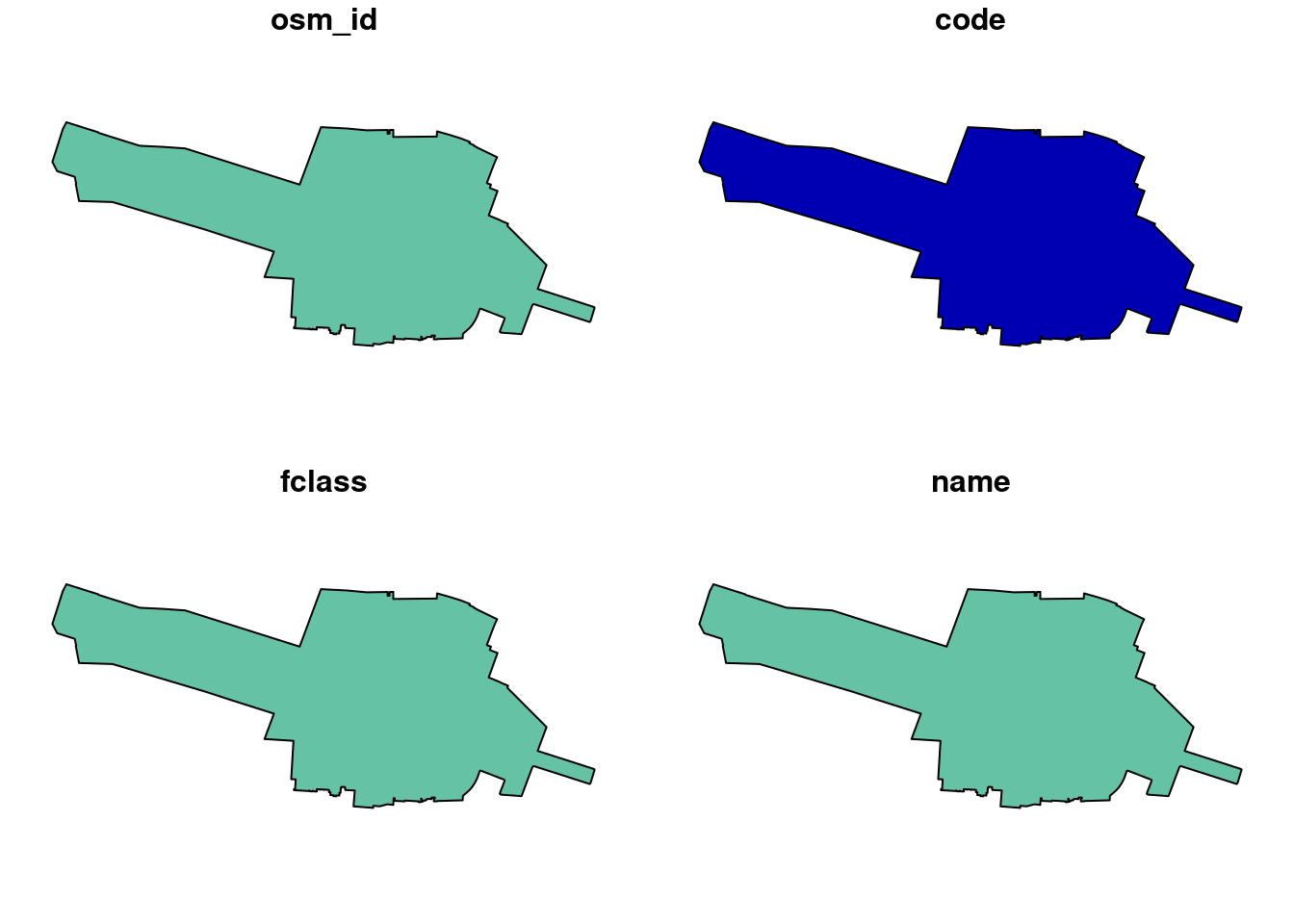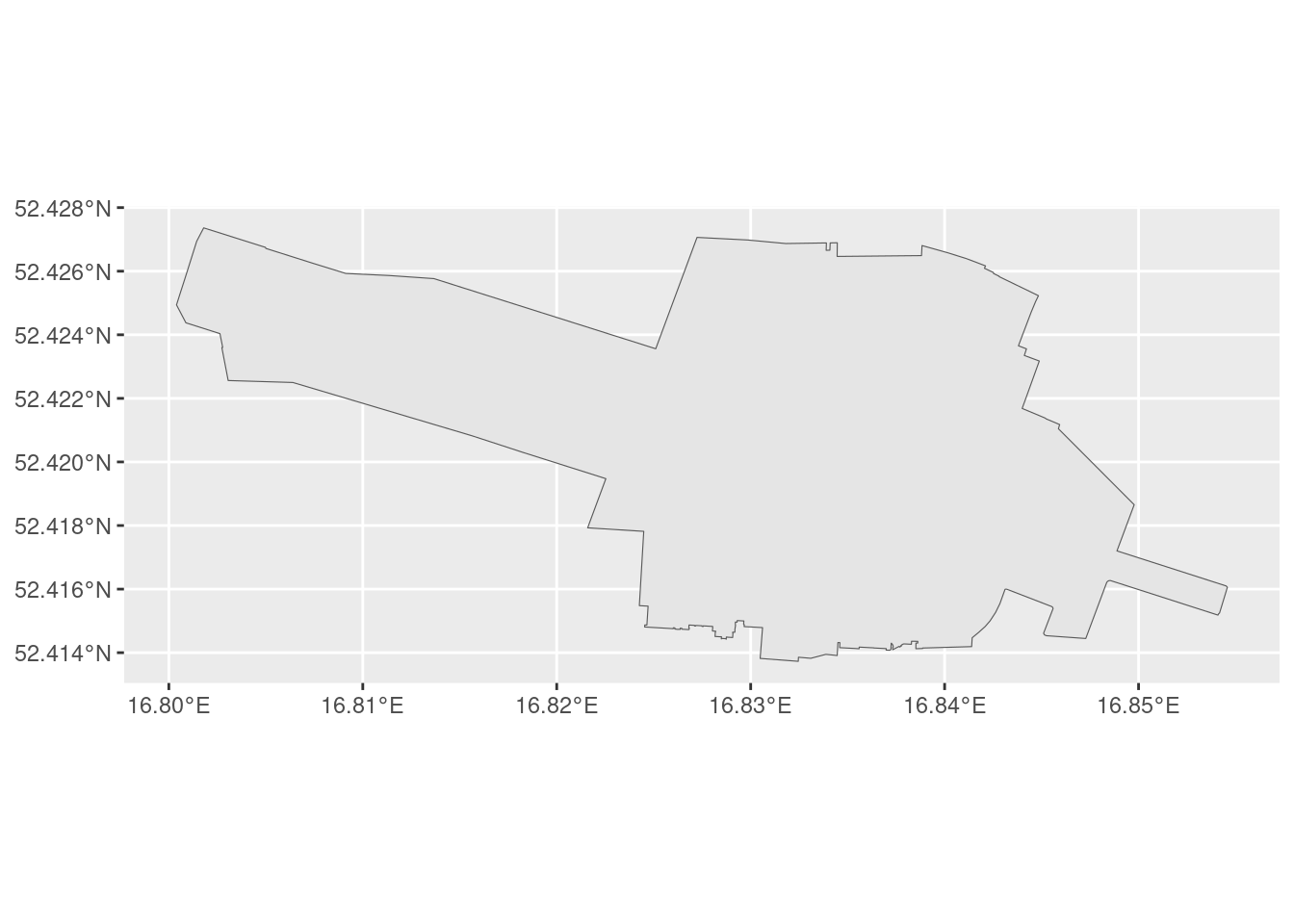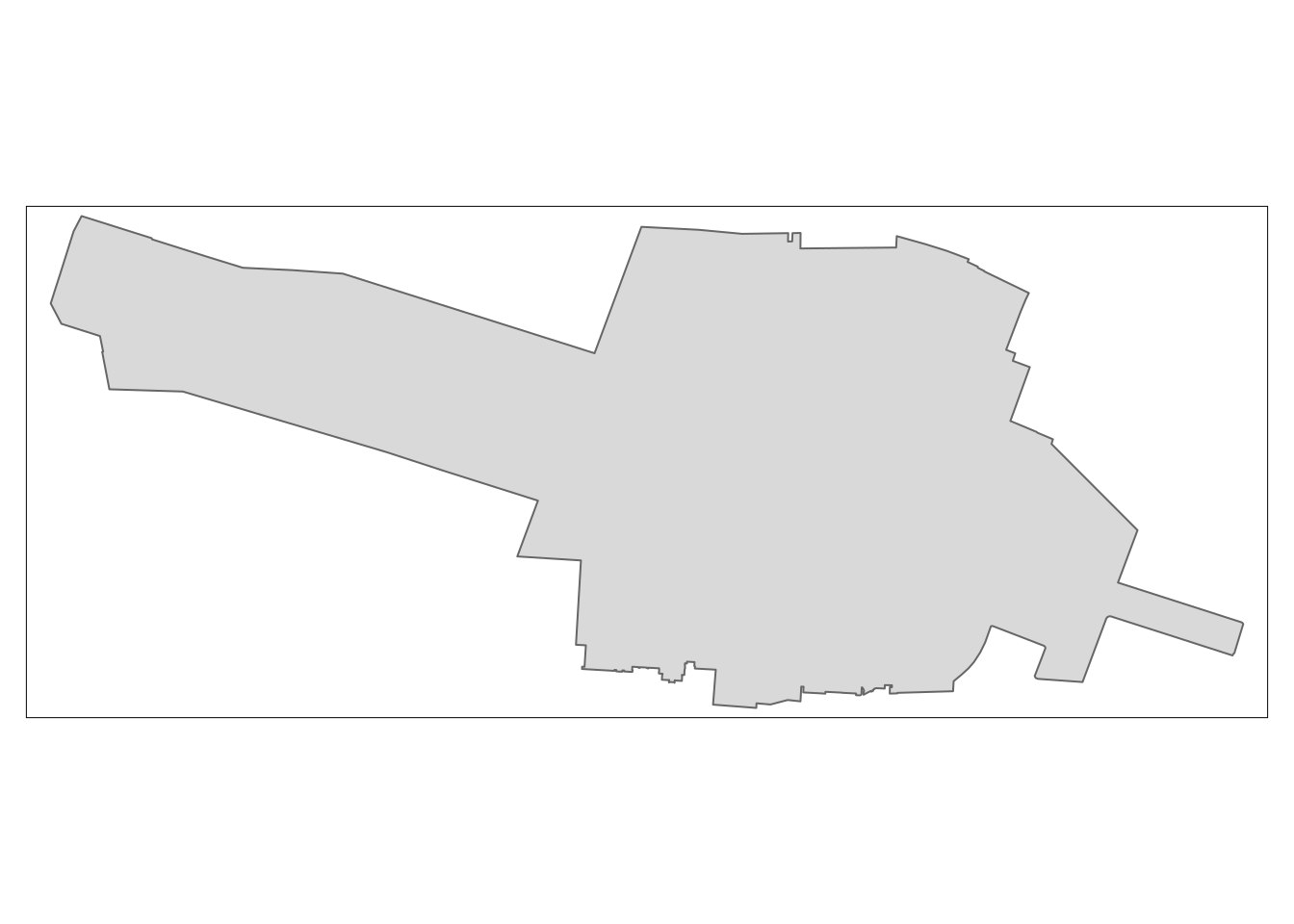We’ll read-in a point layer from a CSV file as shown below.

``stops_raw = read_csv('data/gtfs/stops.txt')``
``````Rows: 2921 Columns: 6
── Column specification ────────────────────────────────────────────────────────
Delimiter: ","
chr (3): stop_code, stop_name, zone_id
dbl (3): stop_id, stop_lat, stop_lon

ℹ Use `spec()` to retrieve the full column specification for this data.
ℹ Specify the column types or set `show_col_types = FALSE` to quiet this message.``````
``````stops_df = stops_raw |>
select(-stop_code)
stops = st_as_sf(stops_df, coords = c("stop_lon", "stop_lat"), crs = "EPSG:4326")``````

### 5.2.1 Buffers

The most widly used way to create buffers in R is with the function `st_buffer()` from the `sf` package. Let’s create buffers of 150 m around each of the points in the `poi_sf` dataset. This is done in the following chunk, which first checks to see if the `s2` spherical geometry engine is set to run (it is by default).

``sf::sf_use_s2()``
`` TRUE``
``poi_buffers = st_buffer(poi_sf, dist = 150)``

As described in Chapter 7 or Geocomputation with R, `sf` ‘knows that the world is round’ and uses a spherical geometry engine to calculate distances for unprojected data. This is a major advantage of `sf` over other packages for working with geographic data, such as `GeoPandas` in Python, which does not currently support spherical geometry operations (see issue 2098 in the GeoPandas issue tracker for details).

We can measure the area of the buffers with the following command:

``areas = st_area(poi_buffers)``

A nice feature of `sf` is that it returns the area in square meters, even though the input data is in degrees. `sf` uses the `units` package behind the scenes to convert between units, meaning you can convert the output to different units, as shown below.

``````areas |>
units::set_units(ha)``````
``````Units: [ha]
 7.165668 7.164428 7.165692 7.166715``````

Sometimes it’s useful to drop the `units` class, which can be done with the `units::drop_units()` function, as shown below.

``````areas |>
units::set_units(ha) |>
units::drop_units() |>
round()``````
`` 7 7 7 7``

### 5.2.2 Spatial subsetting

Note: this section is adapted from Section 2.12 of Working with Spatial Data in Python. Let’s find the bus stops that are within 150 m of the `poi_sf` points.

``````stops_nearby = stops[poi_buffers, ]
stops_nearby``````
``````Simple feature collection with 4 features and 3 fields
Geometry type: POINT
Dimension:     XY
Bounding box:  xmin: 16.92882 ymin: 52.44307 xmax: 16.94161 ymax: 52.4653
Geodetic CRS:  WGS 84
# A tibble: 4 × 4
stop_id stop_name             zone_id            geometry
<dbl> <chr>                 <chr>           <POINT [°]>
1     418 UAM Wydział Geografii A       (16.94108 52.46419)
2     467 Umultowska            A       (16.92882 52.44426)
3     468 Umultowska            A       (16.93039 52.44307)
4     417 UAM Wydział Geografii A        (16.94161 52.4653)``````

### 5.2.3 Spatial joins

Spatial joins can be performed with the `st_join()` function as follows:

``````pois_joined = st_join(poi_buffers, stops)
pois_joined``````
``````Simple feature collection with 6 features and 4 fields
Geometry type: POLYGON
Dimension:     XY
Bounding box:  xmin: 16.92856 ymin: 52.44223 xmax: 16.95195 ymax: 52.46567
Geodetic CRS:  WGS 84
# A tibble: 6 × 5
name                                        geometry stop_id stop_name zone_id
* <chr>                                  <POLYGON [°]>   <dbl> <chr>     <chr>
1 Faculty        ((16.93959 52.46441, 16.93957 52.464…     418 UAM Wydz… A
2 Faculty        ((16.93959 52.46441, 16.93957 52.464…     417 UAM Wydz… A
3 Hotel ForZa    ((16.94759 52.44224, 16.94762 52.442…      NA <NA>      <NA>
4 Hotel Lechicka ((16.93275 52.44435, 16.93275 52.444…     467 Umultows… A
5 Hotel Lechicka ((16.93275 52.44435, 16.93275 52.444…     468 Umultows… A
6 FairPlayce     ((16.9477 52.461, 16.94765 52.46092,…      NA <NA>      <NA>   ``````

### 5.2.4 Exercises

1. Create a static map of the stops in Poznan, using the `stops` object created above, with a mapping package of your preference. Set the colour of each stop by `zone_id`.
• Bonus: also create an interactive map.
2. Advanced: Reproduce the results presented above by following the Python code at geobgu.xyz/presentations/p_2023_ogh/01-vector.html.
• Which language do you prefer for the types of task presented here and why?

## 5.3 Raster data example

Building on the introduction to raster data with Python, this section introduces raster data with the `{terra}` package.

``library(terra)``
``terra 1.7.39``
``````
Attaching package: 'terra'``````
``````The following object is masked from 'package:tidyr':

extract``````

Read-in and plot a single raster layer with the following command:

``````src = rast('data/hls/HLS.S30.T33UXU.2022200T095559.v2.0.B02.tiff')
terra::plot(src, col = gray.colors(10))``````

We will translate the following Python code to R:

``files = glob.glob('data/hls/*.tiff')``
``````files = list.files("data/hls", pattern = "tiff", full.names = TRUE)
files``````
`````` "data/hls/HLS.S30.T33UXU.2022200T095559.v2.0.B02.tiff"
 "data/hls/HLS.S30.T33UXU.2022200T095559.v2.0.B03.tiff"
 "data/hls/HLS.S30.T33UXU.2022200T095559.v2.0.B04.tiff"
 "data/hls/HLS.S30.T33UXU.2022200T095559.v2.0.B08.tiff"``````
``````r = rast(files)
r``````
``````class       : SpatRaster
dimensions  : 3660, 3660, 4  (nrow, ncol, nlyr)
resolution  : 30, 30  (x, y)
extent      : 6e+05, 709800, 5790240, 5900040  (xmin, xmax, ymin, ymax)
coord. ref. : WGS 84 / UTM zone 33N (EPSG:32633)
sources     : HLS.S30.T33UXU.2022200T095559.v2.0.B02.tiff
HLS.S30.T33UXU.2022200T095559.v2.0.B03.tiff
HLS.S30.T33UXU.2022200T095559.v2.0.B04.tiff
HLS.S30.T33UXU.2022200T095559.v2.0.B08.tiff
names       : Blue, Green, Red, NIR_Broad ``````
``summary(r)``
``Warning: [summary] used a sample``
``````      Blue            Green             Red           NIR_Broad
Min.   :-213.0   Min.   :  15.0   Min.   : -12.0   Min.   :  56
1st Qu.: 202.0   1st Qu.: 435.0   1st Qu.: 313.0   1st Qu.:2088
Median : 319.0   Median : 617.0   Median : 586.0   Median :2469
Mean   : 342.3   Mean   : 623.7   Mean   : 669.2   Mean   :2648
3rd Qu.: 434.0   3rd Qu.: 746.0   3rd Qu.: 955.0   3rd Qu.:3174
Max.   :4508.0   Max.   :5131.0   Max.   :5428.0   Max.   :6092  ``````

We can plot the result as follows:

``plot(r)``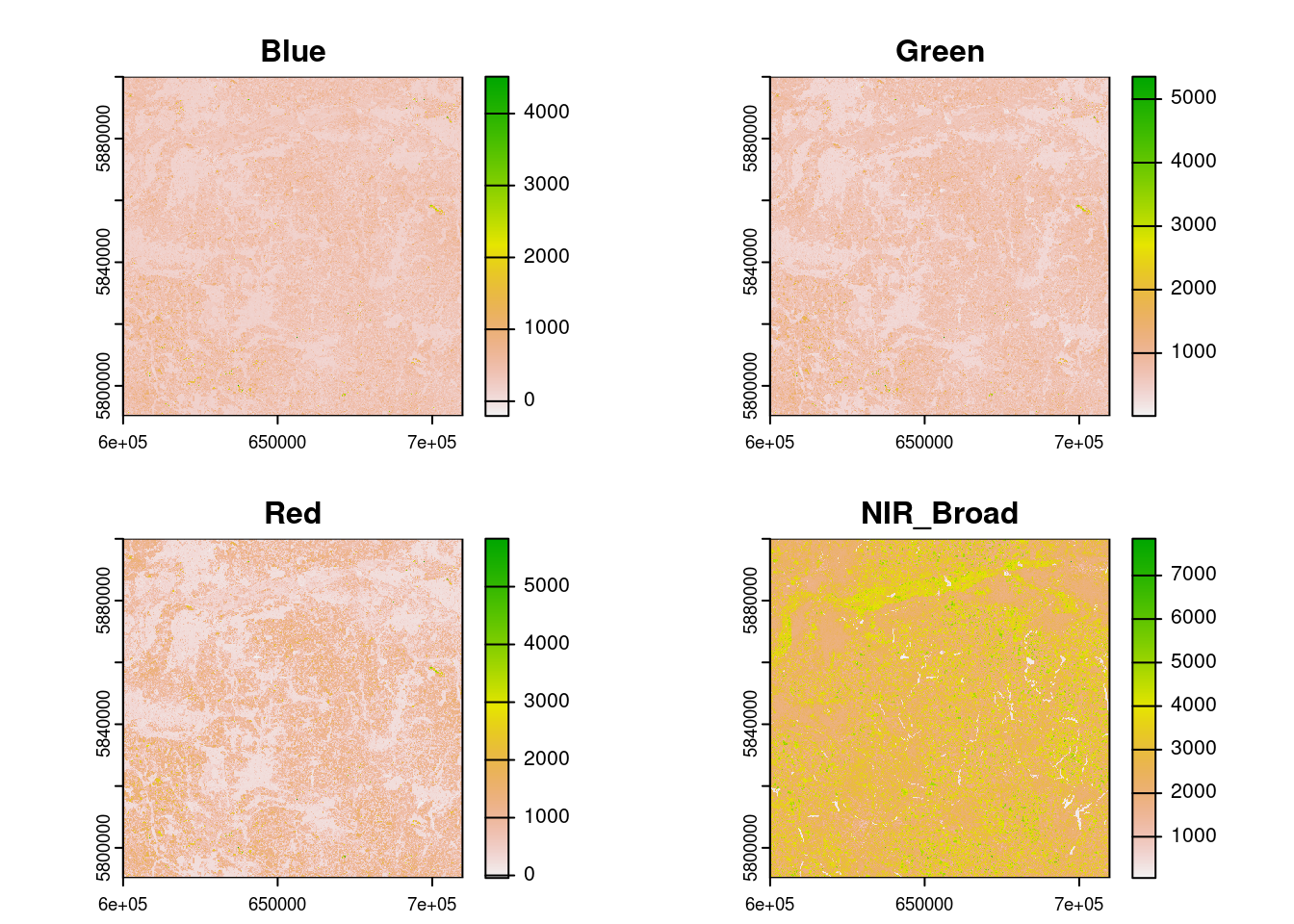Figure 8: Output of plot(r), showing the four bands of the raster layer

As shown, the result is an object with Blue, Green, Red and NIR bands, in that order. We can select only Red, Green, Blue bands, in that order, as follows:

``r_rgb = r[[c("Red", "Green", "Blue")]]``

If you try plotting the result with `plotRGB(r)`, you will get an error. You can use the `stretch` argument of the function to stretch the values and avoid errors caused by outliers.

``plotRGB(r, stretch = "lin")``

We can also remove outliers with the `stretch()` or `clamp()` functions or manually, as shown below:

``````# r_clamp = clamp(r, 0, 4000)
r_stretch = stretch(r_rgb, minq = 0.001, maxq = 0.999)
top_01pct = quantile(values(r_rgb), probs = 0.999, na.rm = TRUE)
bottom_01pct = quantile(values(r_rgb), probs = 0.001, na.rm = TRUE)
r_to_plot = r_rgb
r_to_plot[r_rgb > top_01pct] = top_01pct
r_to_plot[r_rgb < bottom_01pct] = bottom_01pct``````Figure 10: Plotting a RGB raster layer with terra, with outliers handled with stretch() (left) and manually (right)

Save the combined raster as follows:

``````# write the r file:
writeRaster(r, "data/hls/combined.tif", overwrite = TRUE)
writeRaster(r_to_plot, "data/hls/r_to_plot.tif", overwrite = TRUE)``````

We can mask the raster with the `pol` polygon object as follows:

``````pol_projected = sf::st_transform(pol, crs = crs(r))
``````
|---------|---------|---------|---------|
=========================================
``````
``summary(r_masked)``
``Warning: [summary] used a sample``
``````      Blue            Green             Red           NIR_Broad
Min.   : 351.0   Min.   : 623.0   Min.   : 739.0   Min.   :1165
1st Qu.: 482.0   1st Qu.: 744.8   1st Qu.: 803.0   1st Qu.:2136
Median : 526.0   Median : 775.0   Median : 892.0   Median :2338
Mean   : 578.1   Mean   : 843.7   Mean   : 963.0   Mean   :2228
3rd Qu.: 569.2   3rd Qu.: 821.2   3rd Qu.: 985.2   3rd Qu.:2485
Max.   :1618.0   Max.   :2016.0   Max.   :2231.0   Max.   :2709
NA's   :100467   NA's   :100467   NA's   :100467   NA's   :100467  ``````

As shown in the summary of the result, the majority of the values are now NA. That’s how masking works: it sets all values outside the polygon to NA. We can crop the raster to a 500 m buffer around the polygon as follows:

``r_cropped = crop(r, sf::st_buffer(pol_projected, dist = 500))``

Let’s plot the result, illustrated in Figure 11.

``````tm_shape(stretch(r_cropped[[c("Red", "Green", "Blue")]], minq = 0.001, maxq= 0.98)) +
tm_rgb() +
tm_shape(pol_projected) +
tm_borders(col = "white", lty = 3) +
tm_fill(col = "red", alpha = 0.1) +
tm_scale_bar(bg.color = "white", bg.alpha = 0.6, text.size = 0.8) ``````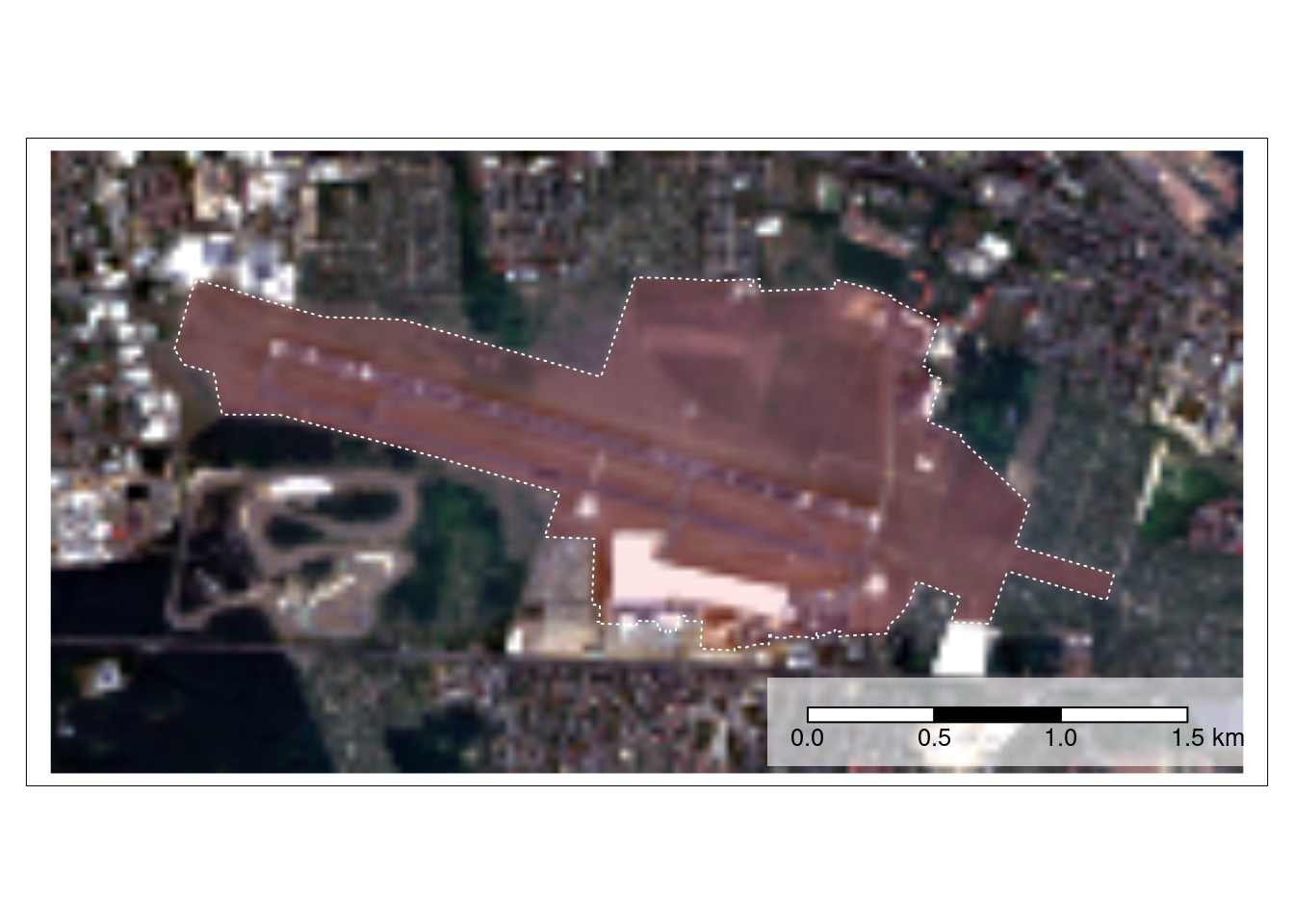Figure 11: Result of plotting a cropped RGB raster layer with the tmap package

### 5.3.2 Exercises

1. Experiment with arguments passed to `clamp()`, `stretch()` and `plotRGB()` to see how they affect the output.
2. Try plotting the raster in another program like QGIS, which looks better?
3. Advanced: Reproduce the results presented above by following the Python code at geobgu.xyz/presentations/p_2023_ogh/02-raster.html.
• Which language do you prefer for the types of task presented here and why?
4. Advanced: Try plotting the raster with `{ggplot2}` and `{tmap}`.
• Which do you prefer and why?

# 6 Other tidy packages

There are many packages that integrate with the `tidyverse`, or which are inspired by it. Options include:

How to assess which to use? My advice would be to focus on solving the problem, rather than the tools, in which case the `tidyverse` + `sf` + `terra` or `stars` should be more than adequate for most purposes. There can be major time savings made by sticking to established tools that are well documented and which have a large user base.

Developer time is more valuable than computer time, so a major factor in deciding which tool to use should be how reliable it is. Time spent debugging issues can be a major time sink, especially for new packages that are not yet well tested or documented. And for most purposes, the tools outlined in the previous sections are more than adequate, solving perhaps 90% of the problems you’re likely to encounter.

There are times when performance is important but as the saying goes, premature optimisation is the root of all evil, so don’t worry about performance until you have a working solution.

If you do need to wait longer than is feasible for your code to run, it is worth trying to identify the bottleneck (is there are a solution involving changing input datasets or workflows?) before having a look at alternatives. Established benchmarks for non-spatial data are https://duckdblabs.github.io/db-benchmark/ and https://www.pola.rs/benchmarks.html .

This section is offered more as a place to experiment than any concrete advice. My concrete advice is focus on the problem and solving it with the software is most appropriate for your use case.

## 6.1`tidypolars`

If you want to give this package a spin, run the following command:

``````install.packages(
'tidypolars',
repos = c('https://etiennebacher.r-universe.dev/bin/linux/jammy/4.3', getOption("repos"))
)``````

## 6.2`rsgeo`

A work in progress is the `rsgeo` package, which aims to provide an seamless interface between R and the `geo` Rust crate. This could open the possiblity of calling other high-performance Rust libraries from R, although the package is at an early stage of development and probably not ready for production use.

We can check the installation works as follows:

``install.packages('rsgeo', repos = c('https://josiahparry.r-universe.dev', 'https://cloud.r-project.org'))``
``````library(rsgeo)
countries_rs  = as_rsgeo(sf::st_geometry(countries_projected))
countries_rs
bench::mark(check = FALSE,
sf = sf::st_union(countries_projected),
geos = geos::geos_make_collection(geos::geos_unary_union(countries_geos)),
rsgeo = rsgeo::union_geoms(countries_rs)
)``````

## 6.3 Arrow

``````library(arrow)

# write countries_projected to parquet file:
write_parquet(countries_projected, "data/countries_projected.parquet") # Fails``````

For more on raster data see https://kadyb.github.io/OGH2023/Clustering.html

The following free and open access resources provide a strong foundation for further learning in this space.

• Wickham, Cetinkaya-Rundel, and Grolemund (2023), hosted at https://r4ds.hadley.nz/, is a detailed introduction to the tidyverse

• Lovelace, Nowosad, and Muenchow (2019) provides a wide ranging yet beginner-friendly introduction to using R for geographic analysis

• Pebesma and Bivand (2023), a textbook on spatial data science by the developers of `sf` and other core ‘rspatial’ packages

• Any other suggestions welcome, there’s much more out there!

## References

Lovelace, Robin, Jakub Nowosad, and Jannes Muenchow. 2019. Geocomputation with R. CRC Press. https://r.geocompx.org/.
Pebesma, Edzer, and Roger Bivand. 2023. Spatial Data Science: With Applications in r. CRC Press. https://r-spatial.org/book/.
Wickham, Hadley. 2014. “Tidy Data.” The Journal of Statistical Software 14 (5). https://vita.had.co.nz/papers/tidy-data.pdf.
———. 2019. Advanced r, Second Edition. 2nd edition. Boca Raton: Chapman; Hall/CRC.
Wickham, Hadley, Mine Cetinkaya-Rundel, and Garrett Grolemund. 2023. R for Data Science: Import, Tidy, Transform, Visualize, and Model Data. 2nd edition. O’Reilly Media.